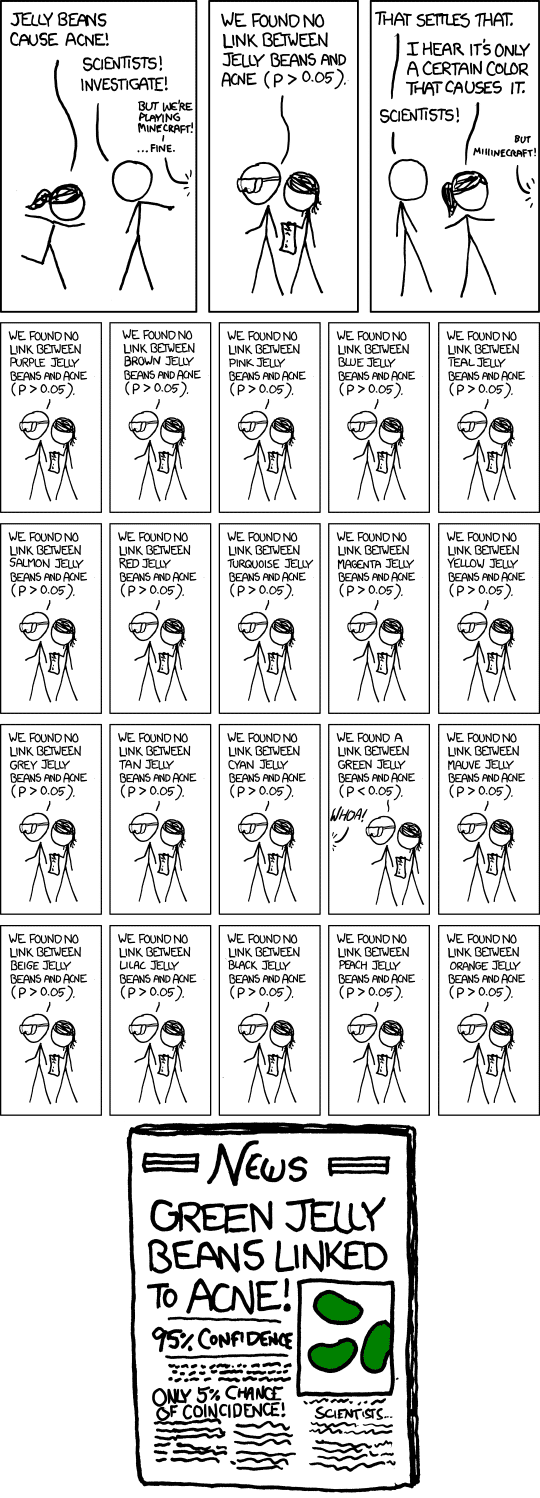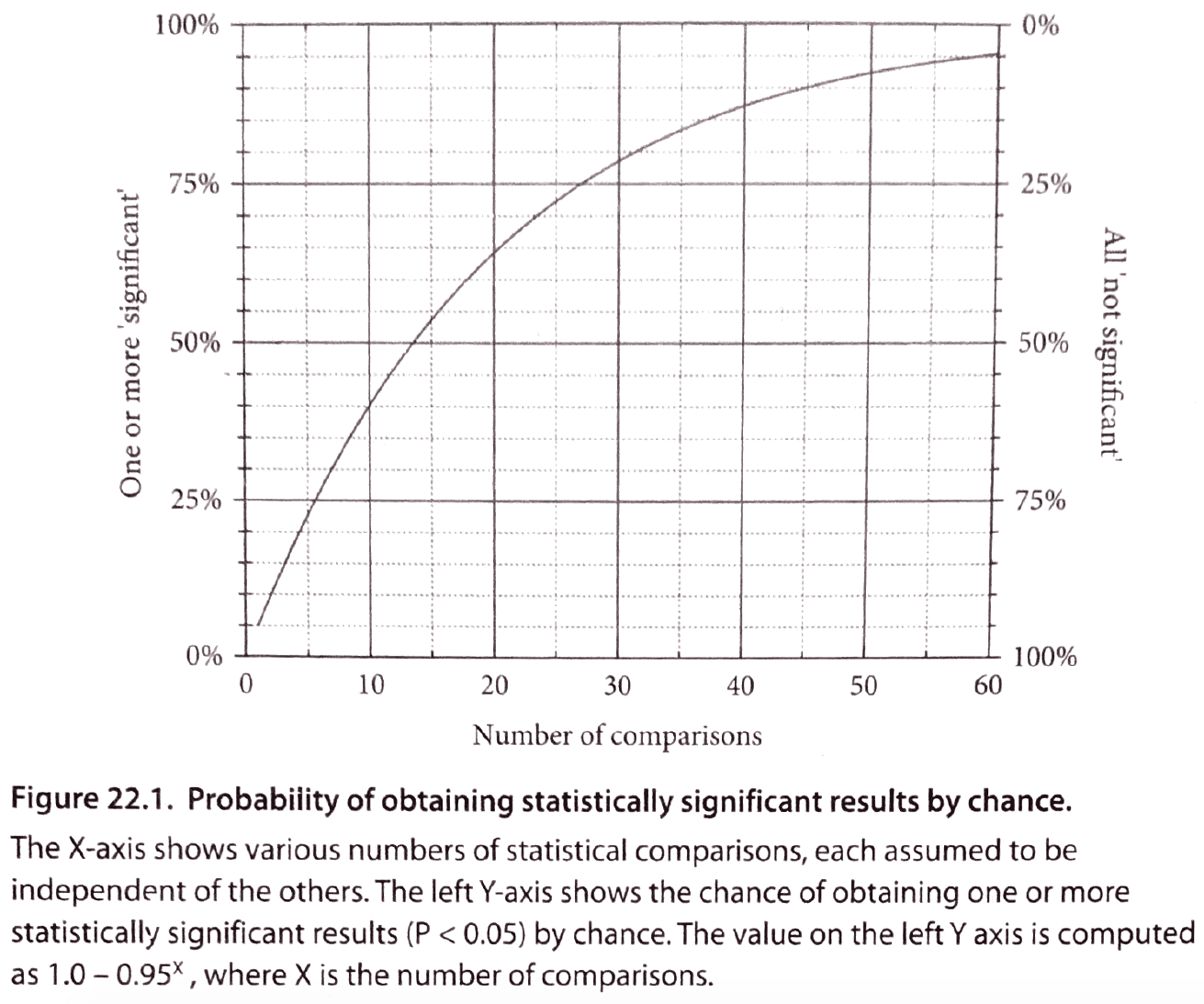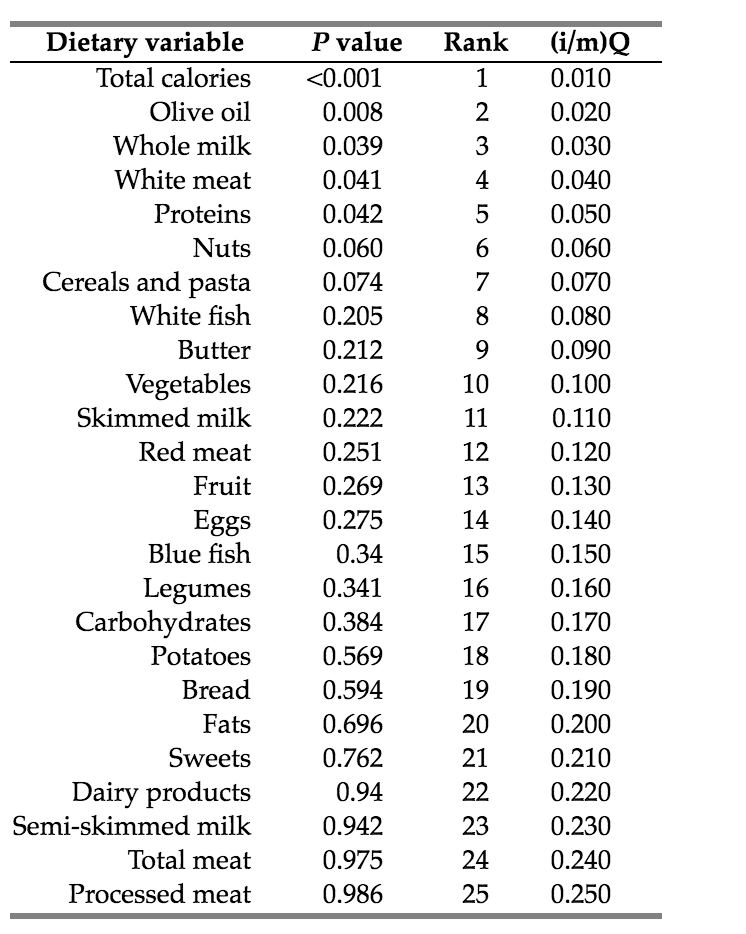# False Positives, FWER, and FDR Explained

If you torture your data long enough, they will tell you whatever you want to hear - Mills (1993)

False positives via statistical hypothesis testing are a severe problem in the scientific literature (Ioannidis, 2005). If a statistically significant finding looks real, but it’s not, and we make policy or clinical decisions based on this finding, it can have catastrophic consequences. Unfortunately, many researchers are still unaware exactly why false positives are so prevalent in the scientific literature, so, I’ve decided to explain some of the common reasons for the high prevalence. But here’s a relevant xkcd comic:Generally, when going with a frequentist statistical approach, we are thinking in the long term. And in the long run, when guiding our behavior via automation, we are willing to tolerat no difference between groups. By convention, most researchers are willing to tolerate that 5% of the time their significant results could be a total fluke, a result that is pure noise.

So, let’s say there is no difference between group A and B who receive different treatments (which have no difference). And we ran a test to compare their change in outcome averages over time; we would falsely conclude that there is a difference between the groups in 5 experiments out of 100 (not really 100, more like 5% of infinity) when there never was an actual difference.

## Independent Comparisons

### The Per-Comparison Error Rate

The long-term error rate is fixed when we make one comparison, also known as the per-comparison error rate. When we begin to make several comparisons, our probability of getting a significant result begins to change.

With one comparison, our probability of getting a false significant result in the long run is 5%, and our probability of getting a nonsignificant result is 95% (1 - .05).

With two comparisons, our probability changes in the following way: the probability of NOT getting a significant result (a nonsignificant result) for one comparison is 95% (0.95), as stated before, and the probability of NOT getting a significant result for the second comparison is ALSO 95% (0.95), it’s the same. We multiply these probabilities (0.95 x 0.95 = 0.9025), which is around 90%. So, with TWO comparisons, the probability of us getting a nonsignificant result is 90%, and the probability of getting at least one false significant result is now 10% (1 - 0.9025 = 0.10).

### The Familywise Error Rate

With more and more comparisons, this number (the false-positive rate) continues to increase in the overall family of comparisons. This is also referred to as the familywise error rate or per-experiment error rate, which, again, is the total number of comparisons run in the study (Hochberg & Tamhane, 1987). The general formula to figure out the probability of getting at least one false significant result as a function of the number of comparisons we make is $$1 - 0.95^k$$, where k is the number of comparisons.

If there is no actual effect and we ran ten independent comparisons, the probability of us getting at least one false significant result is 40%. With 13 independent comparisons, it’s 50%, and with 20 comparisons, it’s 64%. That’s a high probability of finding a significant result that could be pure noise. Controlling these error rates is incredibly essential for making valid statistical inferences.Before I get into a discussion of correcting for multiple comparisons, I want to mention some other areas where multiplicity is a problem.

• Choosing numerous sample sizes until statistical significance is achieved instead of using a method like sequential data analysis

• Using ambiguous primary outcomes and changing them

• Running multiple subgroup analyses

• Preprocessing the data in various ways

• Using multiple analyses until significance is achieved

• Using automatic variable selection in multiple regression (all-subsets regression, forward-stepwise selection, backward-stepwise selection) (Motulsky, 2014)

## Correcting for Multiple Comparisons

### When Not to Correct for Multiple Comparisons

Generally, many statisticians believe that there is no need to adjust for multiple comparisons when testing hypotheses if the following are done:

• If all the p-values are listed for every comparison, and it’s explicitly stated that multiple comparisons have been made, allowing the reader to judge the results for him/herself

• If one of the outcomes has been strictly defined as being a primary outcome and the others are secondary outcomes or exploratory analyses

• If only some of these comparisons were planned stringently with little ambiguity.

Now that we’ve discussed some scenarios where it may not be necessary to correct for multiple comparisons, we can talk about some general approaches to correct for multiple comparisons.

### Per-Comparison Error Rate vs. Familywise Error Rate

As said before, the more comparisons we run, the higher our probability in the long run of getting a false positive. What started off a 5% probability of finding a false significant result begins to skyrocket to nearly 50% by 13 comparisons in the entire family of comparisons.

And this is important to clarify. The probability of us getting a false significant result in the long run per comparison is 5%, meaning that once we look at one p-value, it’s considered significant when under 5%. The per-comparison error rate is fixed. But the more comparisons we run, the higher the long-run probability of obtaining at least one false significant result in the family of comparisons or familywise-error rate. These explanations may seem a bit repetitive, but I believe they’re essential to repeat because the topic is a difficult concept to grasp at first.

### The Bonferroni Correction

One of the oldest and simplest ways to correct for multiple comparisons is to use the Bonferroni correction, named after Italian mathematician Carlo Emilio Bonferroni (Bonferroni, 1936).

The Bonferroni correction seeks to set the familywise error rate back to 5% in the overall family of comparisons from the overall increase that was a result of increased comparisons. It sets it back by dividing the original significance level by the number of comparisons.

So, if we set our significance level to 5% (per comparison error rate) and we ran 13 comparisons which make our familywise error rate 50%, the Bonferroni correction sets the familywise error rate back to 5% by taking the significance level, 5% and dividing it by the number of comparisons, which is 13.

So, that would be 0.05/13= ~0.004. That means for an individual p-value to be significant, it must be under this new threshold (0.004), which is far lower than the original 5% threshold for individual significance. And now, our overall familywise error rate is back to 5%.

A problem with this approach is that it often lowers statistical power (the probability of correctly rejecting the null hypothesis), and the procedure is very conservative. It can reduce the probability of getting a false positive, at the cost of increasing the probability of a false negative. Some modifications have been made to this procedure such as the Holm-Bonferroni correction, which gives us more statistical power (Holm, 1979).

### The Holm-Bonferroni Correction

The Holm-Bonferroni correction takes the original alpha level and divides it by the total number of comparisons subtracted by the rank of the p-value plus one. For each p-value, we would take our alpha level, say, 0.05, divided by the number of total comparisons made, which is 10, and subtract it by the rank of our p-value and then add one.)

So, if we got 10 p-values (0.0001, 0.003, 0.01, 0.04, 0.07, 0.11, 0.14, 0.30, 0.50, 0.60), we would rank them in order of significance, with the smallest as being the highest ranked, as I’ve done. And then we would apply the formula to create a new threshold for each p-value. If the p-value falls under this individualized threshold, it’s significant, if not, then it’s not significant.

Let’s use the smallest ranked p-value,0.0001 as an example. Our original alpha level is 0.05. The number of total comparisons is 10. The rank of the p-value is 1 (the smallest). Plugged into our formula, 0.05 / (10 - 1 + 1) = 0.005 which is our new threshold. Our p-value, 0.0001, is smaller than this new threshold, so it’s significant!

We repeat these steps for each p-value. So for the second-ranked smallest p-value, our formula is 0.05 / (10 - 2 + 1) = 0.005, which is our new threshold. Our p-value, 0.003, is slightly smaller, so it is significant. Let’s try our third p-value, 0.01.

0.05 / (10 - 3 + 1) = 0.006 is our new threshold. 0.01 does not fall under this threshold. Therefore, it is not significant.

Pretty neat, eh?

### The False Discovery Rate

The false discovery rate is an alternative approach to procedures that attempt to control the familywise error rate. First proposed by Yoav Benjamini and Yosef Hochberg in the 1990s (Benjamini & Hochberg, 1995), it focuses on all of the significant values that have been found, referred to as “discoveries,” and attempts to control for the rate of false positives in the overall discoveries made.

This contrasts with the familywise error rate procedures which attempt to control for the number of false positives in all of the comparisons that have been made.

Let’s say we made 100 comparisons. In our familywise error rate approach, we would be thinking about all 100 comparisons and control for false positives out of all these comparisons. With the false discovery rate, we care mainly about the false significant findings (discoveries) out of the significant results in total, rather than all the findings (significant + nonsignificant) in total.

So in an FWER approach, we may try to limit ourselves to 50 false positives out of 1000 comparisons. In an FDR approach, we might restrict ourselves to a 10% false discovery rate (chosen by the researcher). Let me further unpack this. Say out of the 1000 comparisons, only 50 were significant, with an FDR approach we focus mainly on these 50 significant findings (discoveries) and we want 10% to be false positives, so 5 to be false positives.

This approach generally tries to address two questions:

• If a finding is found to be significant (a discovery), what is the probability that there is no effect?

• Out of all of the significant findings (discoveries), what proportion of them are false discoveries?

### The Benjamini Hochberg FDR Method

In the Benjamini Hochberg method, we rank all of the p values, in a similar way to the Holm-Bonferroni method, from smallest to largest. So, the lowest p-value would have a rank of 1 and so on.

And then we calculate a critical value for each p-value from this formula ((i/m)Q) where i is the rank of the p-value, m is the total number of comparisons made, and Q is the false discovery rate you have chosen (similar to selecting an alpha level).

After we list the p-values along with their critical values, we look for the largest p-value that is smaller than the critical value. Once we have found that p-value, we consider all the p-values lower than it to be significant, even if those small individual p values are not larger than their respective critical values. Here’s an example below.### Alternative Options

Another way to handle multiplicity is to fit a multilevel hierarchical model as the authors have done in the following study and I think this may be worth covering more in a separate blog post because this one is already long enough.

## Concluding Remarks

Multiplicity really is a severe problem in the scientific literature, and it’s not always necessary to correct for multiple comparisons. In fact, some statisticians are highly against it. What we can conclude though is that it is essential to be very open with our procedures, and that as long as we acknowledge that some analyses are exploratory, we can better relay to our readers that some things in the results may just be an artifact of noise.

• Cite this blog post